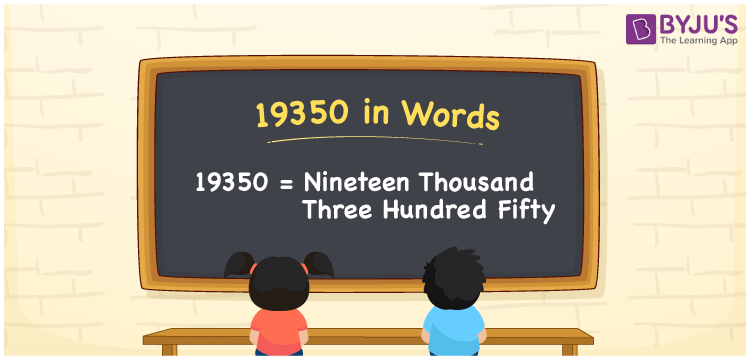# 19350 in words

19350 in words is written as Nineteen Thousand Three Hundred and Fifty. In 19350, 1 has a place value of ten thousand, 9 is in the place value of thousand, 3 has a place value of hundred and 5 is in the place value of ten. The article on Place Value gives more information. The number 19350 is used in expressions that relate to money, distance, Social media views, and many more. For example, “I need at least Nineteen Thousand Three Hundred and Fifty more views for my social media post today”.

 19350 in words Nineteen Thousand Three Hundred and Fifty Nineteen Thousand Three Hundred and Fifty in Numbers 19350

## 19350 in English Words## How to Write 19350 in Words?

We can convert 19350 to words using a place value chart. The number 19350 has 5 digits, so let’s make a chart that shows the place value up to 5 digits.

 Ten thousand Thousands Hundreds Tens Ones 1 9 3 5 0

Thus, we can write the expanded form as:

1 × Ten thousand + 9 × Thousand + 3 × Hundred + 5 × Ten + 0 × One

= 1 × 10000 + 9 × 1000 + 3 × 100 + 5 × 10 + 0 × 1

= 19350.

= Nineteen Thousand Three Hundred and Fifty.

19350 is the natural number that is succeeded by 19349 and preceded by 19351.

19350 in words – Nineteen Thousand Three Hundred and Fifty.

Is 19350 an odd number? – No.

Is 19350 an even number? – Yes.

Is 19350 a perfect square number? – No.

Is 19350 a perfect cube number? – No.

Is 19350 a prime number? – No.

Is 19350 a composite number? – Yes.

## Solved Example

1. Write the number 19350 in expanded form

Solution: 1 x 10000 + 9 x 1000 + 3 x 100 + 5 x 10 + 0 x 1

Or Just 1 x 10000 + 9 x 1000 + 3 x 100 + 5 x 10

We can write 19350 = 10000 + 9000 + 300 + 50 + 0

= 1 x 10000 + 9 x 1000 + 3 x 100 + 5 x 10 + 0 x 1.

## Frequently Asked Questions on 19350 in words

Q1

### How to write the number 19350 in words?

19350 in words is written as Nineteen Thousand Three Hundred and Fifty.
Q2

### State whether True or False. 19350 is divisible by 3?

True. 19350 is divisible by 3.
Q3

### Is 19350 divisible by 10?

Yes. 19350 is divisible by 10.